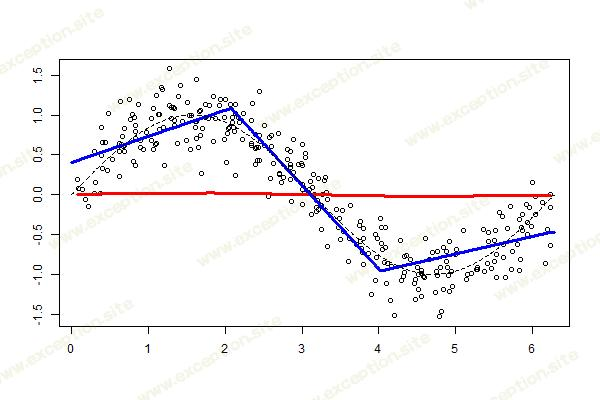# 样条提升的更新

``> library(splines) > fit=lm(y~bs(x,degree=1,df=3),data=df)``

• 从一些回归模型开始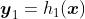• 计算残差，包括一些收缩参数，• 在步骤, 考虑回归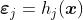• 更新残差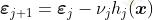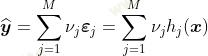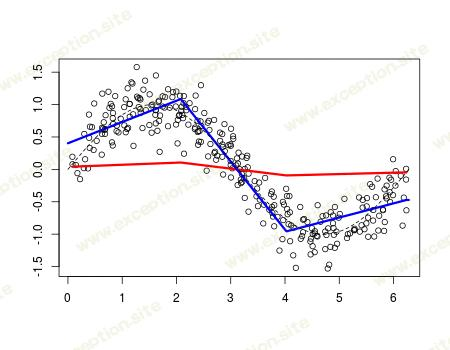``> n=300 > set.seed(1) > u=sort(runif(n)*2*pi) > y=sin(u)+rnorm(n)/4 > df=data.frame(x=u,y=y)``

``> library(freeknotsplines) > xy.freekt=freelsgen(df\$x, df\$y, degree = 1, + numknot = 2, 555)``

``> v=.05 > library(splines) > xy.freekt=freelsgen(df\$x, df\$y, degree = 1, + numknot = 2, 555) > fit=lm(y~bs(x,degree=1,knots= + xy.freekt@optknot),data=df) > yp=predict(fit,newdata=df) > df\$yr=df\$y - v*yp > YP=v*yp > for(t in 1:200){ + xy.freekt=freelsgen(df\$x, df\$yr, degree = 1, + numknot = 2, 555) + fit=lm(yr~bs(x,degree=1,knots= + xy.freekt@optknot),data=df) + yp=predict(fit,newdata=df) + df\$yr=df\$yr - v*yp + YP=cbind(YP,v*yp) + } > nd=data.frame(x=seq(0,2*pi,by=.01)) > viz=function(M){ + if(M==1) y=YP[,1] + if(M>1) y=apply(YP[,1:M],1,sum) + plot(df\$x,df\$y,ylab="",xlab="") + lines(df\$x,y,type="l",col="red",lwd=3) + fit=lm(y~bs(x,degree=1,df=3),data=df) + yp=predict(fit,newdata=nd) + lines(nd\$x,yp,type="l",col="blue",lwd=3) + lines(nd\$x,sin(nd\$x),lty=2)} > viz(100)``